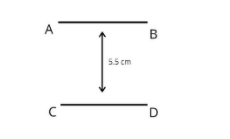QuestionAnswers

# Draw a line CD parallel to the given line AB at a distance of 5.5 cm from it.Verified
128.7k+ views
Hint: A parallel line drawn to the given line using geometry at a certain distance from the line given.

Complete step by step solution:First draw a straight line AB of whichever length. Now, take a compass and draw a ${{90}^{\circ }}$ angle using it at the two end points on the line AB. Even if we draw a single line at ${{90}^{\circ }}$ angle it won’t make much difference. We can also take a protractor and draw a ${{90}^{\circ }}$ angle.
Draw a straight line perpendicular to the line AB through those ${{90}^{\circ }}$ angles. Measure with a scale where you reach 5.5 cm height.
Now, draw a straight line passing from those two straight lines passing through ${{90}^{\circ }}$angles you have drawn from the line AB.
Additional information: A vector having a parallel line (facing the vector with an angle of ${{180}^{\circ }}$) is always equal in value with the given vector. The value here we are talking about is the i, j, k value which makes a vector.
Basically the magnitude of these two parallel vectors should be equal to each other even if they are far apart from each other.

Note: A parallel line from a certain given line can be at any point and direction in the certain distance given to you(here the certain distance was given to be 5.5 cm) but the prerequisite is that both the lines should be facing each other at ${{180}^{\circ }}$. It can also be done using a single straight line of 5.5 cm.